Equivalent Fractions 2
Set 2 (10 Questions)
Students understand that fractions and decimals may be represented by drawings or concrete materials to show equivalency, and to refer to parts of a set and parts of a whole. Students recognize, name, and compare unit fractions from 1/12 to 1/2. Students recognize fractions of a whole and parts of the group, e.g: 1/4 of a pie, or 2/3 of a group of 15 balls. Students know that when all fractional parts are included, such as 4/4, the result is equal to the whole and to one. Students compare fractions represented by drawings or concrete materials.
From Mr. Anker Tests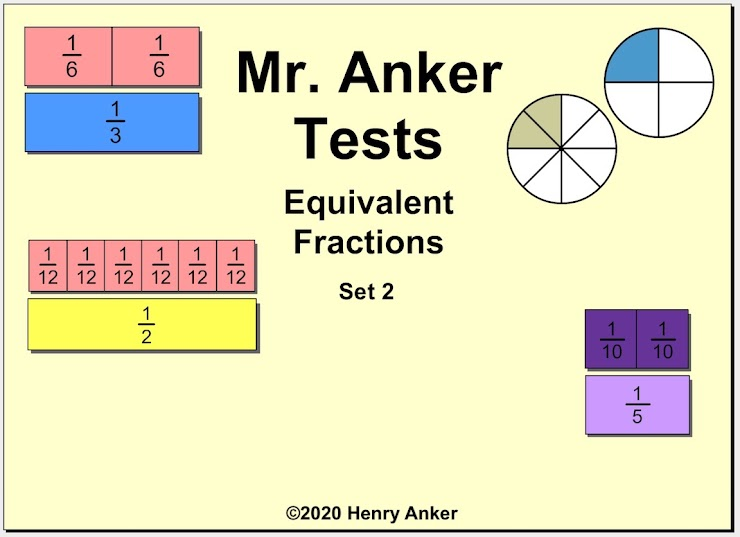Equivalent Fractions Help Slide 1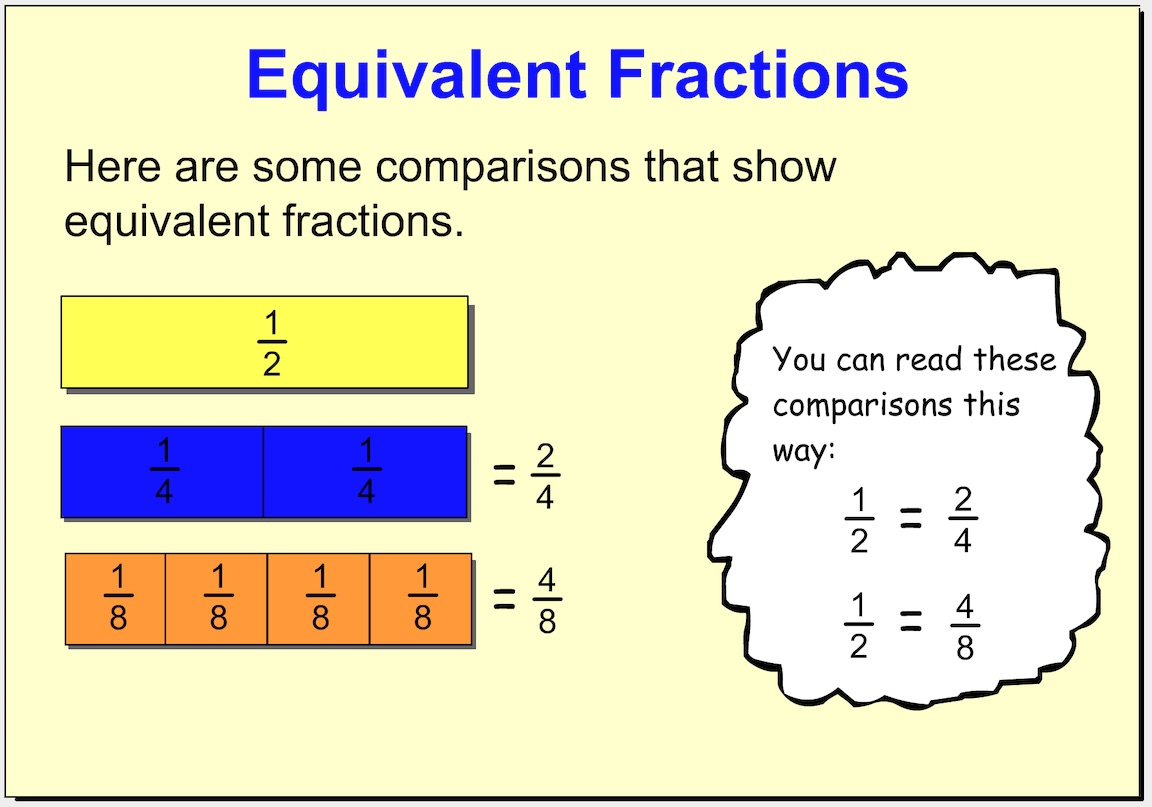Equivalent Fractions Help Slide 2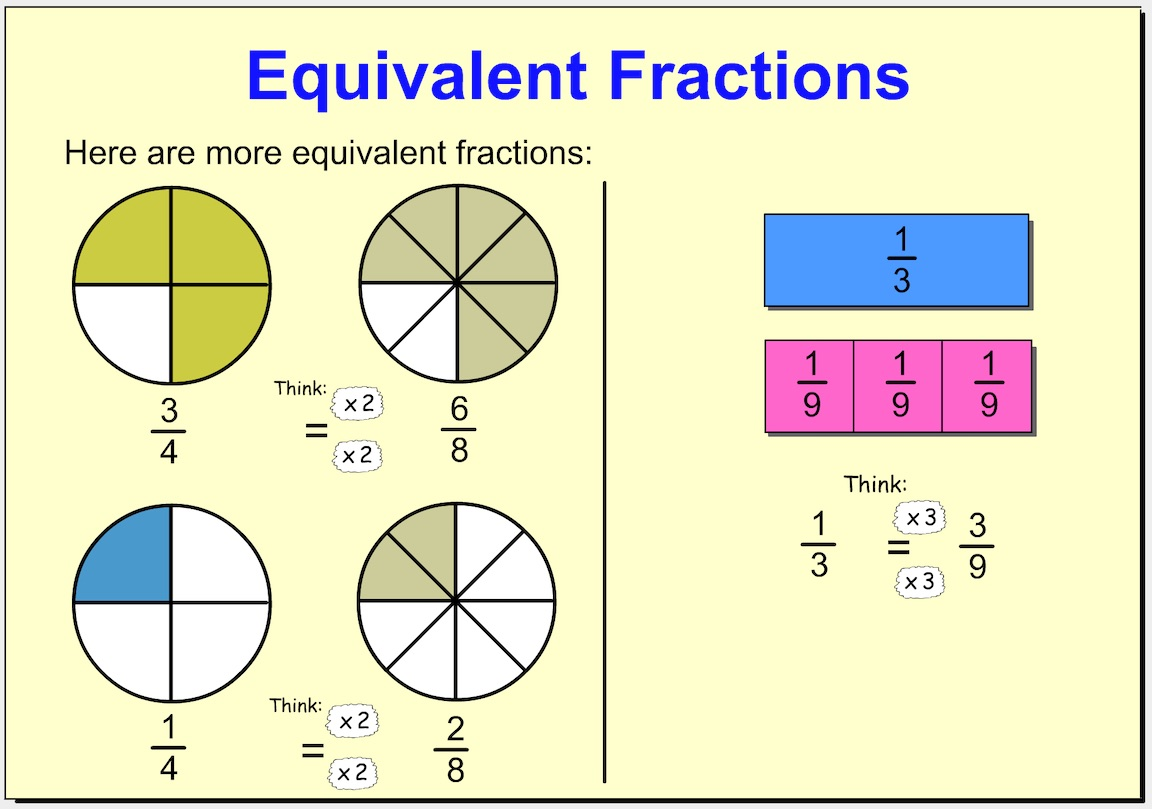Equivalent Fractions Help Slide 3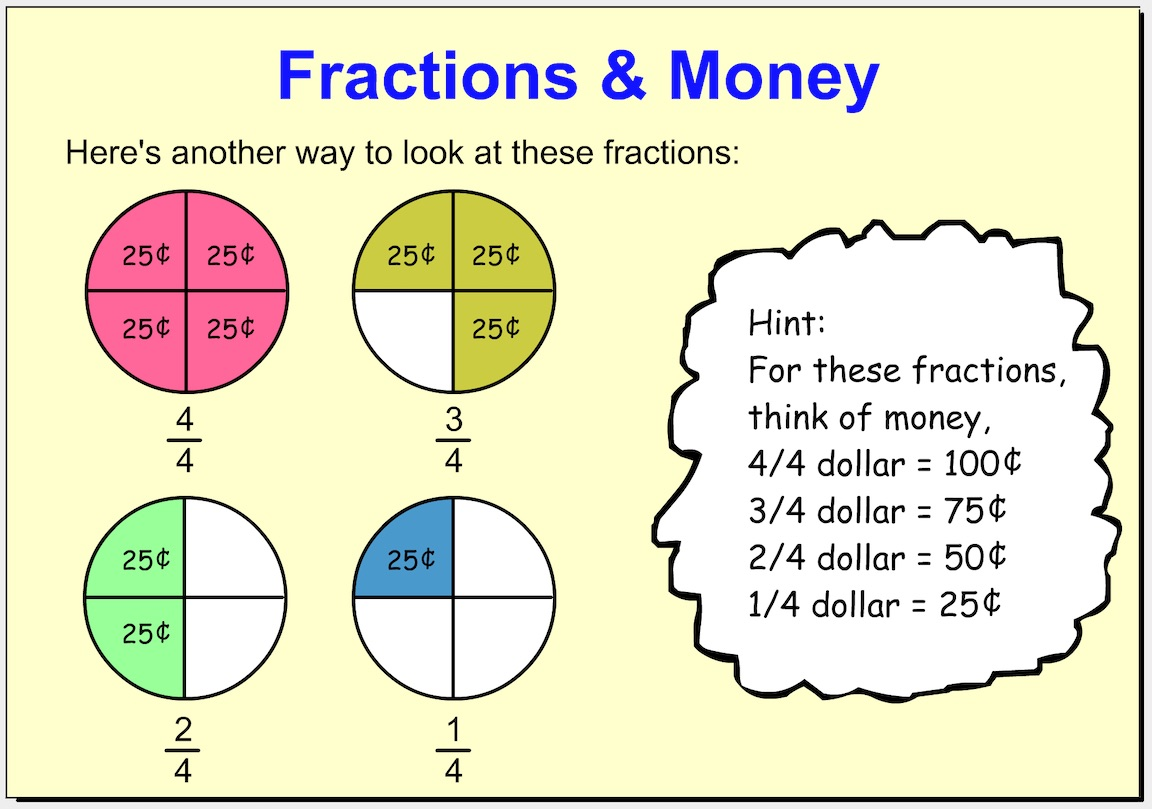1.  Which set of fraction pieces below is equal to 2/3? *
1 point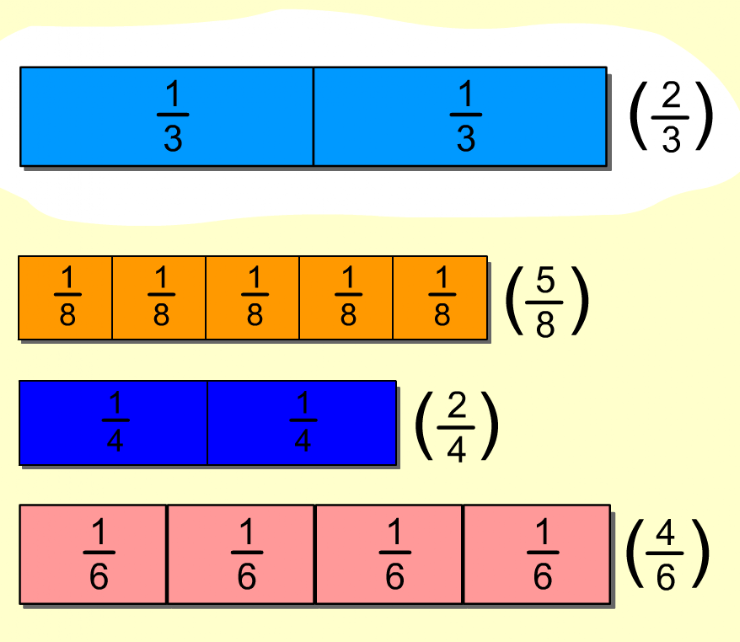2.  Which set of fraction pieces below is equal to 5/10? *
1 point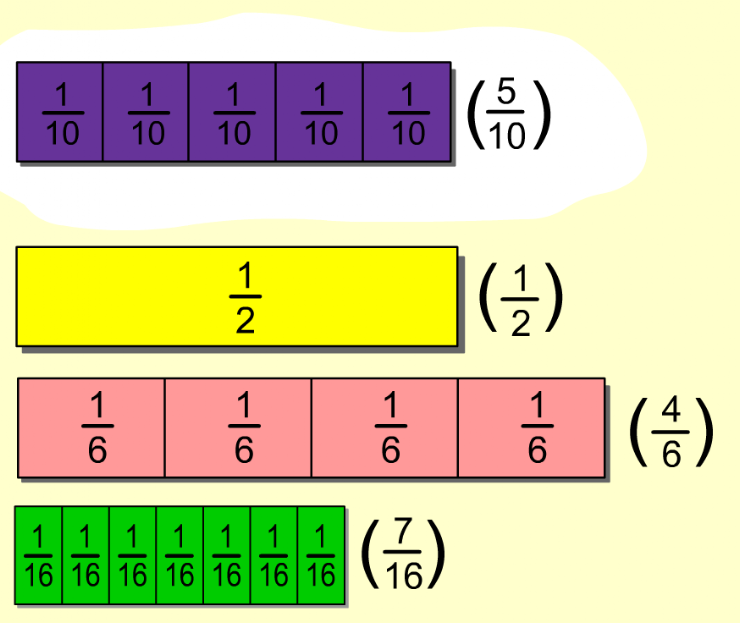3.  Which set of fraction pieces below is equal to 3/8? *
1 point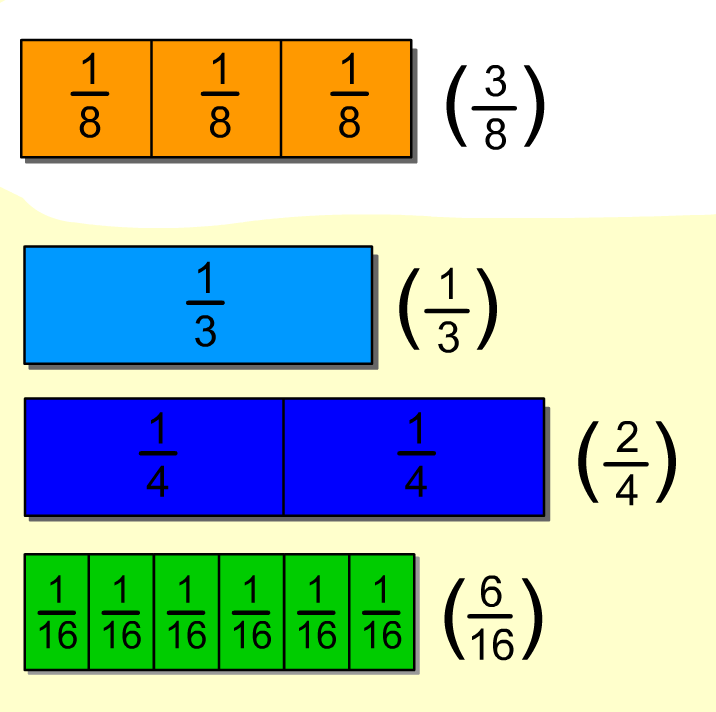4. Which set of fraction pieces below is equal to 3/5? *
1 point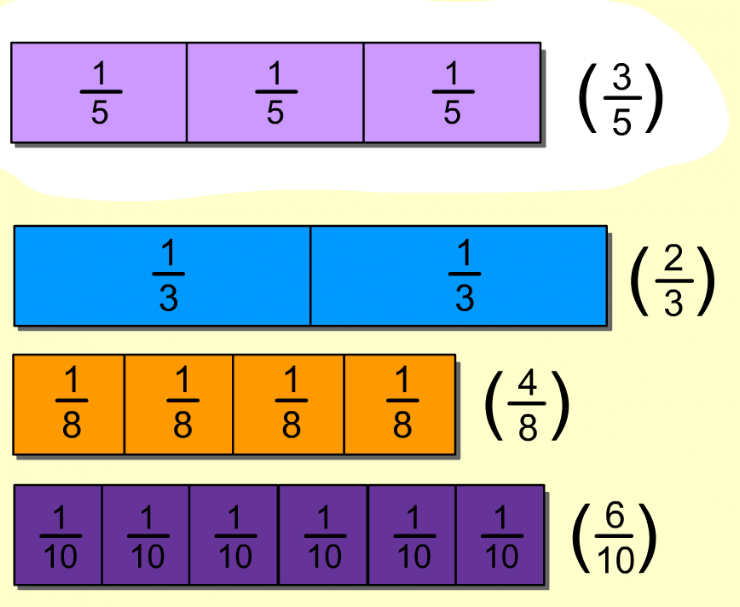5. Which set of fraction pieces below is equal to 5/8? *
1 point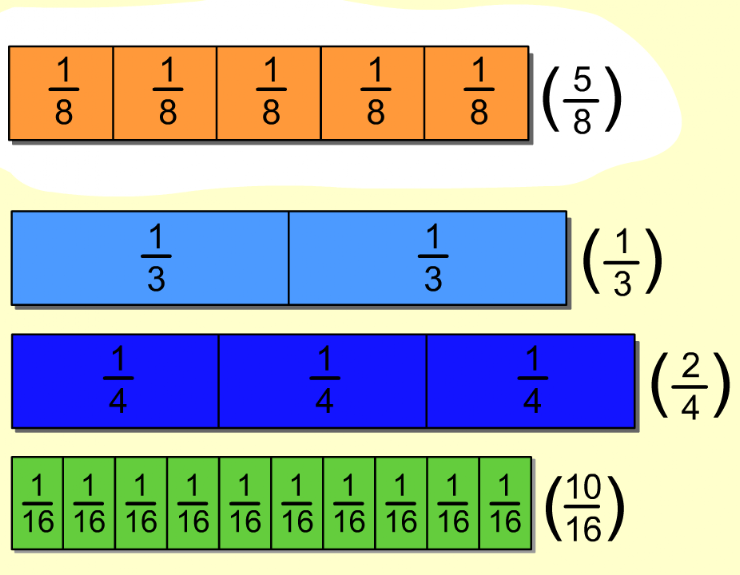6. Which set of fraction pieces below is equal to 2/4? *
1 point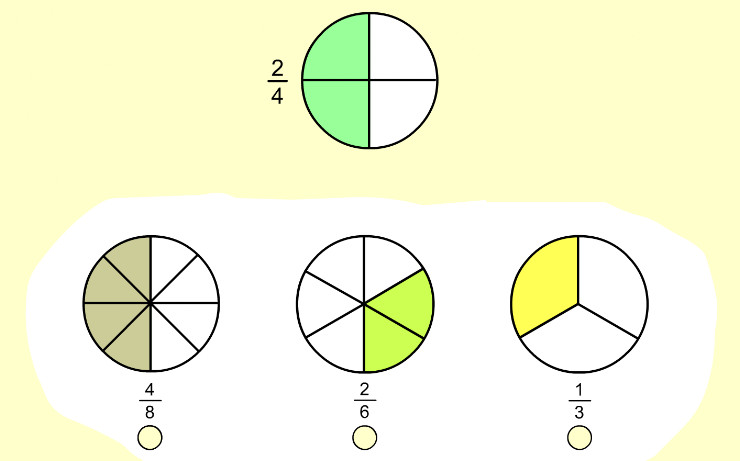7.  Which set of fraction pieces below is equal to 4/6? *
1 point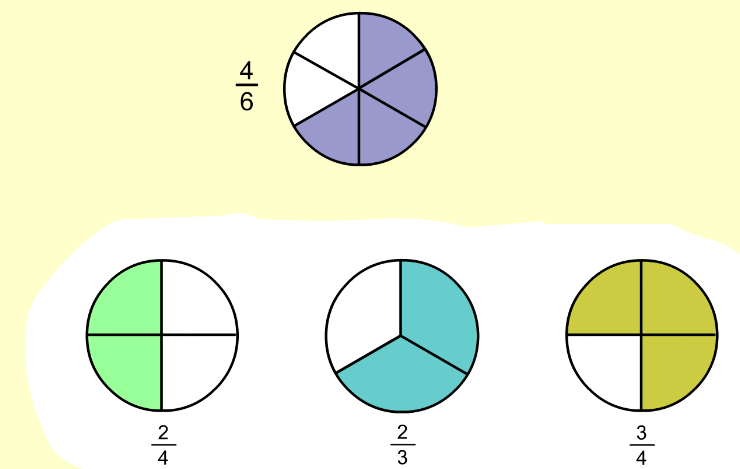8. Which set of fraction pieces below is equal to 3/3? *
1 point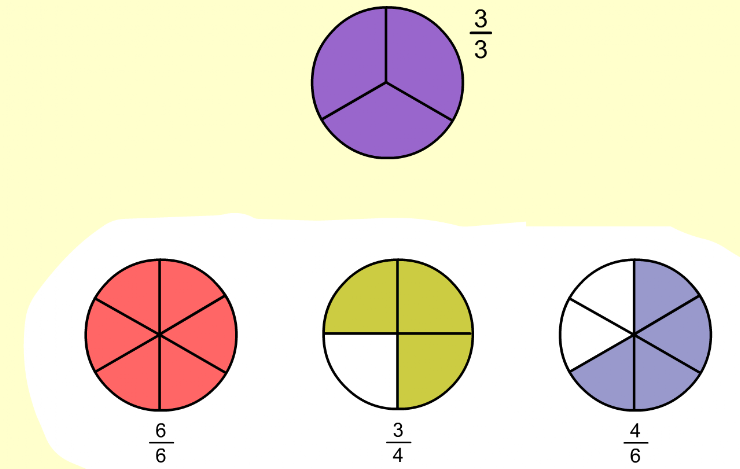9. Which set of fraction pieces below is equal to 1/3? *
1 point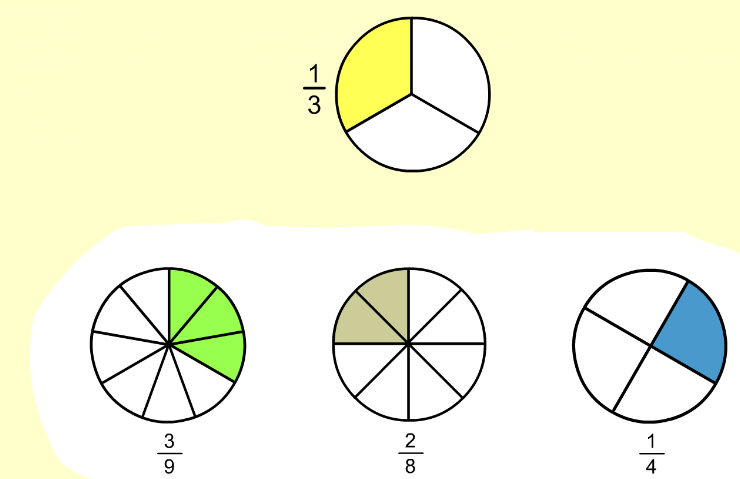10. Which set of fraction pieces below is equal to 1/4? *
1 point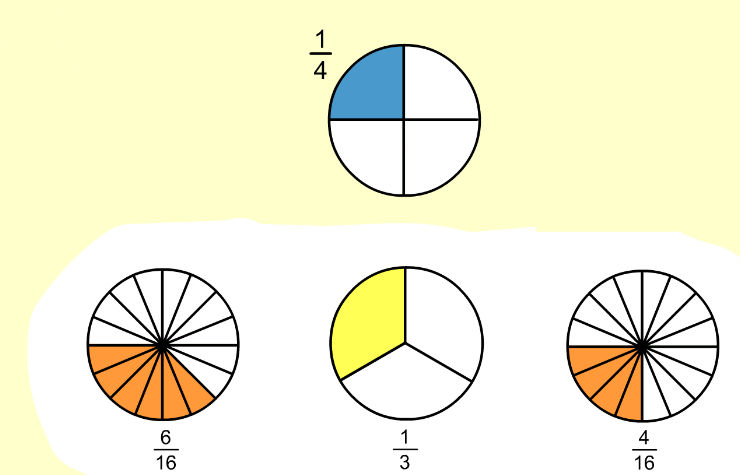Submit
Clear form